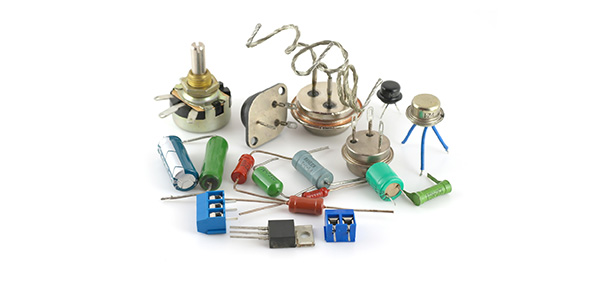# Electronics Quiz

10 Questions | Total Attempts: 36Settings.

Related Topics
• 1.
What is the value of a resistor with the colour bands red, violet, yellow, gold?
• A.

720,00 Ω

• B.

330 Ω

• C.

270,000 Ω

• D.

24,000 Ω

• 2.
How do you identify the positive side of an L.E.D?
• A.

Short leg

• B.

Bent leg

• C.

Long leg

• D.

Two leg

• 3.
What does the minus sign (-) on a capacitor mean?
• A.

It is the dangerous side

• B.

It is the positive side

• C.

It is the negative side

• D.

It is the magnetic side

• 4.
What does is the notation for 1,000 in mathematics?
• A.

Q

• B.

P

• C.

S

• D.

K

• 5.
Where tool is used to remove solder from circuits?
• A.

Solder sucker

• B.

Solder remover

• C.

Solder blower

• D.

Solder eater

• 6.
What is the minimum amount of electrical current deemed lethal?
• A.

0.001A

• B.

0.01A

• C.

0.1A

• D.

1A

• 7.
What do you need to know before reading a circuit diagram?
• A.

The date of the circuit

• B.

What the symbols are

• C.

The nights watch vows

• D.

The time

• 8.
What is the Ultra Violet (UV) box used for?
• A.

Getting a tan

• B.

Designing the circuit board.

• C.

Cooking food

• D.

Burning an image on the circuit board

• 9.
What does the symbol Ω mean?
• A.

Voltage

• B.

Resistance

• C.

Current

• D.

Ground

• 10.
What does L.E.D stand for?
• A.

Low emitting display

• B.

Low energy display

• C.

Light energy diode

• D.

Light emitting diode